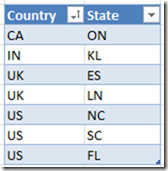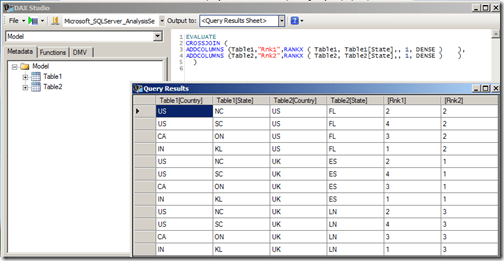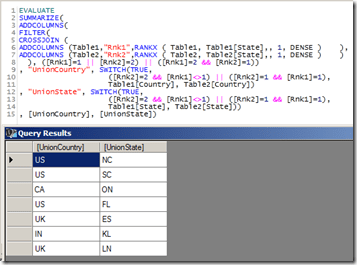# UNION Operation in DAX Queries

In one of my previous posts – Row Selection Using Slicers in PowerPivot – Part 1, I had demonstrated the use of what Marco Russo defined as Reverse Linked Tables and Linkback tables. A particularly eye-catching thing in my post was the use of Microsoft Query instead of DAX Query to obtain the required reverse-linked table and one of the reasons I gave was that it was difficult to do an UNION operation using DAX queries (yes, you heard it right. I said difficult and not impossible). Well, since difficult is such a subjective word, I decided to jot down the technique, maybe it might seem easy for you guys!For the purpose of this demonstration, I am using two tables having identical structures. There are just two columns in the tables – country and state and we need to do an UNION operation on these two tables.The result should be 7 rows as shown belowFollow the steps below for the solution:-

1) Import the two tables to PowerPivot (you can also use this technique on a SSAS tabular model). I will be using DAX Studio for writing my queries and displaying the results (though you might as well as use this in SSMS or in the DMX query editor for SSRS depending on your purpose).

2) The first thing to understand here is that DAX, as a query language, can not add rows usually, and UNION requires that we get more rows (since it is the combined result of both the tables). However, there is an operator in DAX which generally generates more rows than its source tables – CROSSJOIN (except when any one of the participating tables has only one row). So let’s first crossjoin the two tables and see the results.Now you can see that we get 12 rows, however no single column gives the result that we need. Somehow, we need to get a logic to filter the 5 rows and also a logic to combine the right results in one calculated column.

3) To identify the individual rows, let us add a rank column to each of the two tables and then crossjoin them.

EVALUATE
CROSSJOIN (
ADDCOLUMNS (Table1,”Rnk1″,RANKX ( Table1, Table1[State],, 1, DENSE )    ),
ADDCOLUMNS (Table2,”Rnk2″,RANKX ( Table2, Table2[State],, 1, DENSE )    )
)4) When I looked at this resultset initially, I felt that I could just filter all rows having Rank1 = 1 and Rank2 = 2 and then add a calculated column each for Country and State such that if Rank1 = 1, then the value comes from Table2 else it comes from Table1.

EVALUATE
FILTER (
CROSSJOIN (
ADDCOLUMNS (Table1,”Rnk1″,RANKX ( Table1, Table1[State],, 1, DENSE )        ),
ADDCOLUMNS (Table2,”Rnk2″,RANKX ( Table2, Table2[State],, 1, DENSE )        )
),
[Rnk1] = 1 || [Rnk2] = 2    ),
“UnionCntry”, IF ( [Rnk1] = 1, Table2[Country], Table1[Country] ),
“UnionState”, IF ( [Rnk1] = 1, Table2[State], Table1[State] )
)Even though the UnionCntry and UnionState columns might appear right, they are not, as one row is missing (in this case, the row with IN country and KL state). This happens because there will always be one row which has Rnk1 = 1 and Rnk2 = 2, and hence there is an overlap. So we need to think of a different technique to filter the rows.

5) Since we are short of one row, we need to include one more extra row having the complementary values of ranks for the overlapping row (in this case, the row with Rnk1=2 and Rnk2=1). This can be done by using the query given below.

EVALUATE
FILTER(
CROSSJOIN (
ADDCOLUMNS (Table1,”Rnk1″,RANKX ( Table1, Table1[State],, 1, DENSE )    ),
ADDCOLUMNS (Table2,”Rnk2″,RANKX ( Table2, Table2[State],, 1, DENSE )    )
), ([Rnk1]=1 || [Rnk2]=2) || ([Rnk1]=2 && [Rnk2]=1))6) Now all we have to do is to get the logic for creating the calculated columns. We can do it with the help of a SWITCH statement by (a) assigning the extra row (Rnk1=2 and Rnk2=1) to Table1 and (b) making sure that the overlapping row (Rnk1=1 and Rnk2=2) is assigned to Table2. This can be done by the following query

EVALUATE
FILTER(
CROSSJOIN (
ADDCOLUMNS (Table1,”Rnk1″,RANKX ( Table1, Table1[State],, 1, DENSE )    ),
ADDCOLUMNS (Table2,”Rnk2″,RANKX ( Table2, Table2[State],, 1, DENSE )    )
), ([Rnk1]=1 || [Rnk2]=2) || ([Rnk1]=2 && [Rnk2]=1))
, “UnionCountry”, SWITCH(TRUE,
([Rnk2]=2 && [Rnk1]<>1) || ([Rnk2]=1 && [Rnk1]=1)
,Table1[Country], Table2[Country])
, “UnionState”, SWITCH(TRUE,
([Rnk2]=2 && [Rnk1]<>1) || ([Rnk2]=1 && [Rnk1]=1)
,Table1[State], Table2[State]))Now you can see that the UnionCountry and UnionState column gives us the required results for a UNION ALL operation. This approach can be easily extended in case we have more than two tables to join. The only scenario where this approach will not work is when any of the participating tables has just one row (as the cross join will not give us more rows. Eg, cross-joining two tables with 3 and 1 rows will give only 3 rows whereas we need 4 rows for the UNION ALL). However, this can be resolved by adding a temporary table with two rows (so the cross join will be 3 * 1 * 2 = 6 rows) and then using this logic.

7) To do the UNION operation instead of UNION ALL, we should remove all duplicate rows. For that, we can use SUMMARIZE function.

EVALUATE
SUMMARIZE(
FILTER(
CROSSJOIN (
ADDCOLUMNS (Table1,”Rnk1″,RANKX ( Table1, Table1[State],, 1, DENSE )    ),
ADDCOLUMNS (Table2,”Rnk2″,RANKX ( Table2, Table2[State],, 1, DENSE )    )
), ([Rnk1]=1 || [Rnk2]=2) || ([Rnk1]=2 && [Rnk2]=1))
, “UnionCountry”, SWITCH(TRUE,
([Rnk2]=2 && [Rnk1]<>1) || ([Rnk2]=1 && [Rnk1]=1),
Table1[Country], Table2[Country])
, “UnionState”, SWITCH(TRUE,
([Rnk2]=2 && [Rnk1]<>1) || ([Rnk2]=1 && [Rnk1]=1),
Table1[State], Table2[State]))
, [UnionCountry], [UnionState])In this scenario, there is no difference in the result as there are no duplicate rows. Let me know what you think of this!

Update – 11/20/2015

I still see some hits on this page, so just wanted the readers to be aware that DAX now supports the UNION function (along with other set based function) out of the box

UNION Function DAX### Posted by SQLJasongbrueckl

Hey Jason,
thats really an interesting topic, so I also did some tests and came up with this solution:

EVALUATE
SUMMARIZE(
CROSSJOIN(
SUMMARIZE(
Table1,
ROLLUP(ROLLUPGROUP(Table1[Country], Table1[State])),
"IsSubTotalTable1",
ISSUBTOTAL(Table1[Country])),
Table2),
"CountryUnion",
IF([IsSubTotalTable1], Table2[Country], Table1[Country]),
"StateUnion",
IF([IsSubTotalTable1], Table2[State], Table1[State])),
[CountryUnion],
[StateUnion])

first add a SubTotal-row for Table1
then do a CrossJoin with Table2
whenever we are on SubTotal of Table1, we take the values from Table2, otherwise we use the values from Table1
finally use SUMMARIZE to get nly the distinct values

what do you think?Unknown

At first sight, the solution provided by Gerhard could be faster.
But now that I think about that, why not using GENERATE instead of SUMMARIZE? The second argument of GENERATE is executed inside a row context defined by the first argument. If the first argument is a SUMMARIZE with a sub-total row, you could use such a test to conditionally generate only the rows of TABLE2, returning a single ROW in all the other cases… this should be better for memory and performance…Unknown

(previous comment written by Marco Russo)SQL_Jason

Sorry guys, this page had around 9 more comments where Gerhard, thedataspecialist and myself were discussing this technique. Those got deleted by mistake and looks like there is no way to recover it ( I was able to recover all the main comments, but not the replies attached to it). My apologies!Scott

I gave this a try by using a tally table to create the rows for the base table, i added a one column table called tally with the numbers 1-20 (obviously you would need enough values to cover your table sizes).

I'm not sure how the dax engine would actually execute this query, this might not scale if the addcolumns/rankx is build and disposed for every row.

evaluate
filter(tally,tally[row]<=(countrows(table1)+countrows(table2)))
,"country",
if(tally[row]<=countrows(table1),
)
,"state",
if(tally[row]<=countrows(table1),
)
)
order by [country],[state]Tony

Hi,
Whether this query can be used to create tables inside the tabular model in SSAS tabular model?SQL_JasonTony

This is very helpful thank you very much!!!!Hi Jason,

Thank you very much for this!! Your solution has saved my day.

SELECT cust_name AS Customer from cust_table
UNION
SELECT "ALL" AS Customer
order by CustomerGot the solution!! you can use below DAX query as table1 and cust_table as table2 and crossjoin the tables(Follow solution explained by "gbrueckl" above comment)

summarize(row("customer", "ALL"),rollup(rollupgroup([customer])),"Is sub Total", ISSUBTOTAL([customer]))Raja

Thank you. That did help a lot. I wanted a default of ‘NA’ in my parameter drop down. This solved that issue. The only change that I did is to use GENERATEALL instead of CROSSJOIN as CROSSJOIN will not give any rows if the table2 has no rows. Thanks much…mark bombyk

When I do the first Crossjoin at the beginning of the exercise

evaluate
CROSSJOIN(Table1,Table2)

I get the following mssg:

A column named 'Country' already belongs to this Data Table.

What am I doing wrong?mohan vijay

CROSSJOIN(Table1,Table2) is resulting in a single row.
but the SQL Union all will results two rows 1 from table 1 and other from table 2 .

Need Some DAX function that works exactly as
Select city from country
union all
select city from mytableSQL_Jason

DAX now has a UNION operation – https://msdn.microsoft.com/en-us/library/dn802530.aspx That will retain the duplicate row also 🙂

[…] the only problem is the UNION function that was also added in the 2016 version. Jason Thomas wrote an article on simulating the UNION operation in Excel 2013 that might be of […]Priya1234

How do i make @Salesperson multi valued parameter optional in a report using shared dataset.The parameter gets populated from-
evaluate
values(‘SalesPeople'[SALESPERSON_NAME])
order by ‘SalesPeople'[SALESPERSON_NAME]

I looked at crossjoin and many ideas,but dont know how to write it out in dax.I tried to union with “Null” but dont know the DAX for it.My SSRS wont support union because it is an older version.The shared dataset query has filter like the where clause of sql-
PATHCONTAINS (
SUBSTITUTE (
SUBSTITUTE ( SUBSTITUTE ( @Salesperson, “{ “, “” ), ” }”, “” ),
“&”,
“|”
),
‘SalesPeople'[SALESPERSON_NAME]
)
I dont know how to code for “if the @Salesperson parameter is optional”.Pls helpterry hull

Hi, I thought union was to be the answer however it has a serious trap , in that if you are using it on other tables where you may amend the formula that created a field it will re-order the original table, which means that your union statement is not no longer valid. the only work around ive found is to re-create the table as a brand new one and then redo the union statement. hopefully somewhere someone knows a trick to manually set table column orders.

This site uses Akismet to reduce spam. Learn how your comment data is processed.